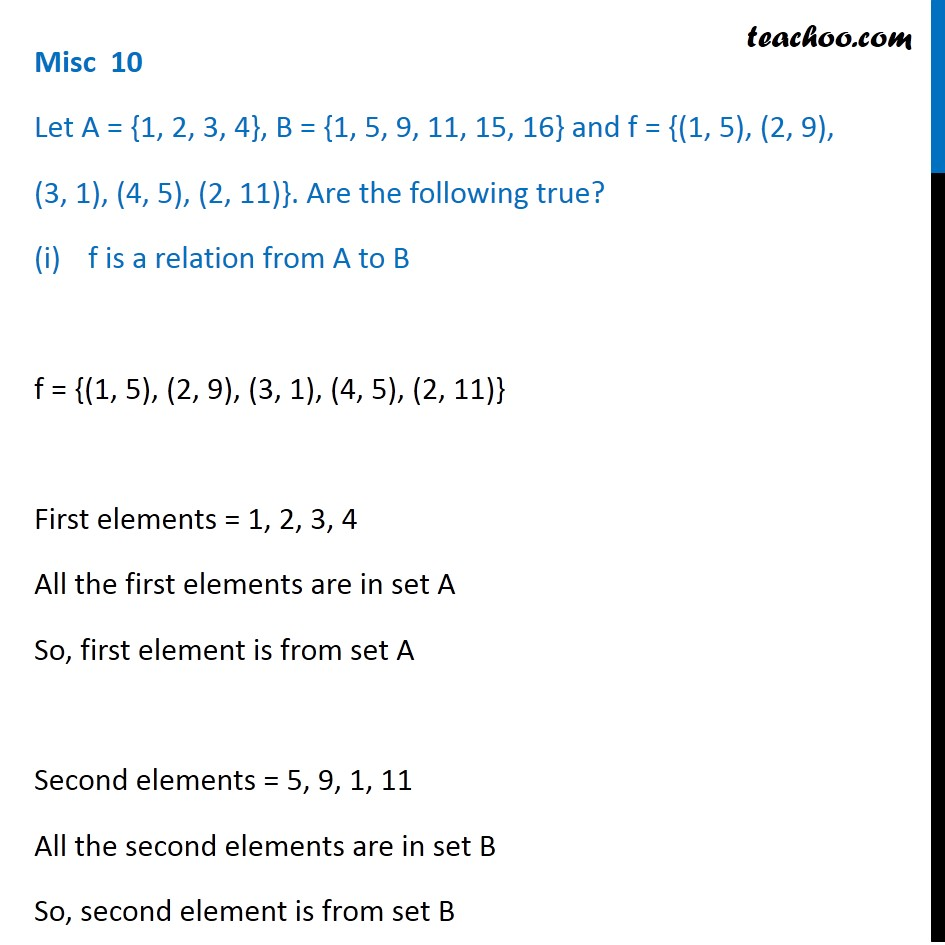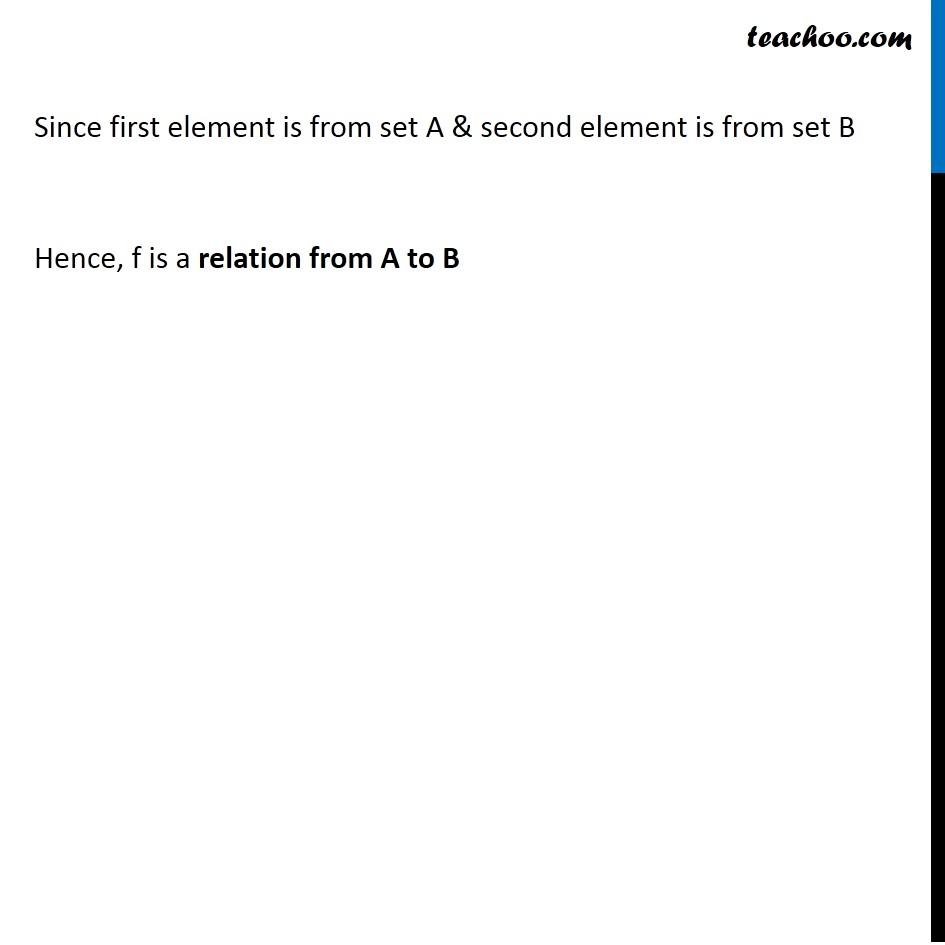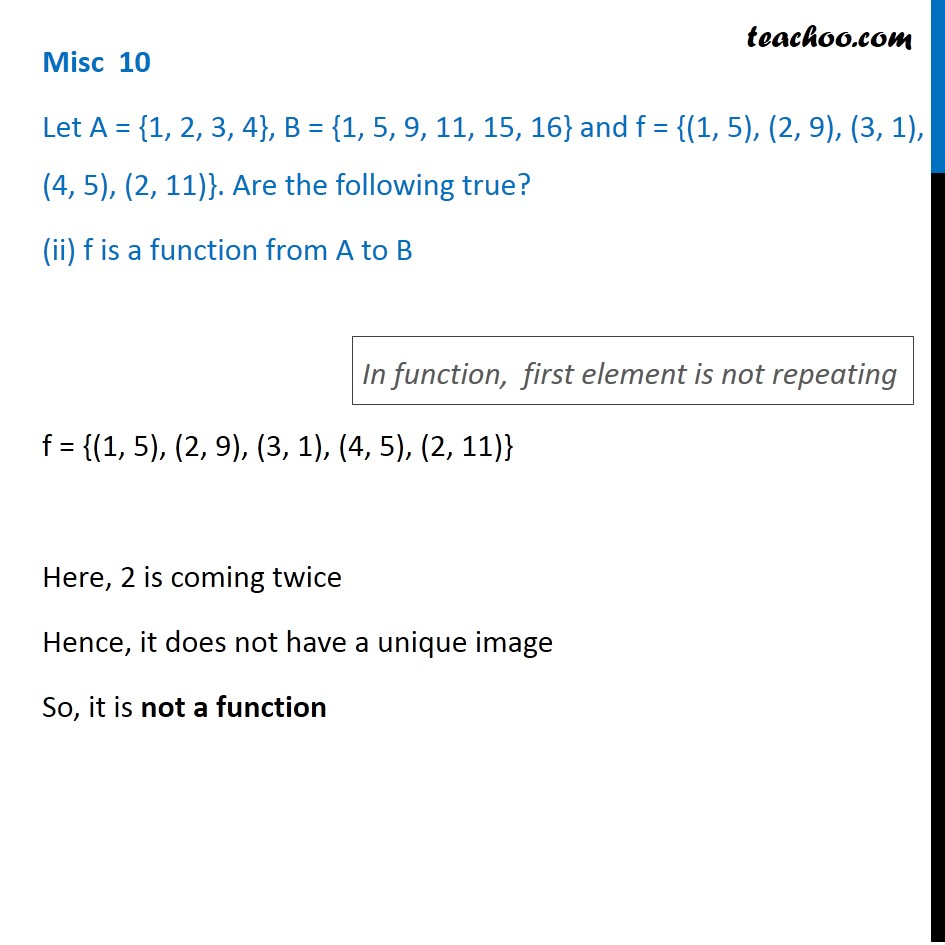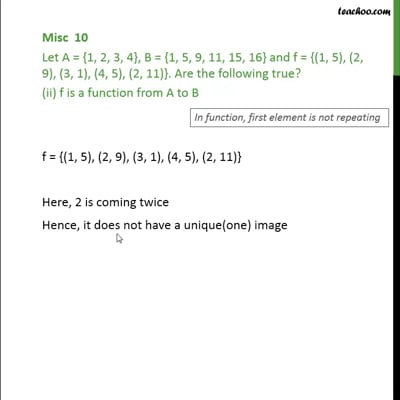Miscellaneous

Chapter 2 Class 11 Relations and Functions (Term 1)
Serial order wiseThis video is only available for Teachoo black users

### Transcript

Misc 10 Let A = {1, 2, 3, 4}, B = {1, 5, 9, 11, 15, 16} and f = {(1, 5), (2, 9), (3, 1), (4, 5), (2, 11)}. Are the following true? f is a relation from A to B f = {(1, 5), (2, 9), (3, 1), (4, 5), (2, 11)} First elements = 1, 2, 3, 4 All the first elements are in set A So, first element is from set A Second elements = 5, 9, 1, 11 All the second elements are in set B So, second element is from set B Since first element is from set A & second element is from set B Hence, f is a relation from A to B Misc 10 Let A = {1, 2, 3, 4}, B = {1, 5, 9, 11, 15, 16} and f = {(1, 5), (2, 9), (3, 1), (4, 5), (2, 11)}. Are the following true? (ii) f is a function from A to B f = {(1, 5), (2, 9), (3, 1), (4, 5), (2, 11)} Here, 2 is coming twice Hence, it does not have a unique image So, it is not a function In function, first element is not repeating